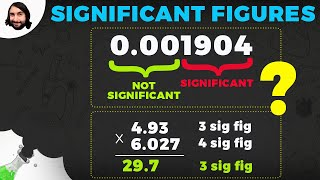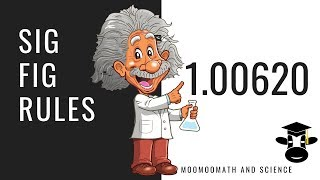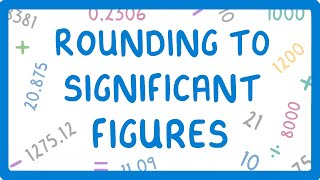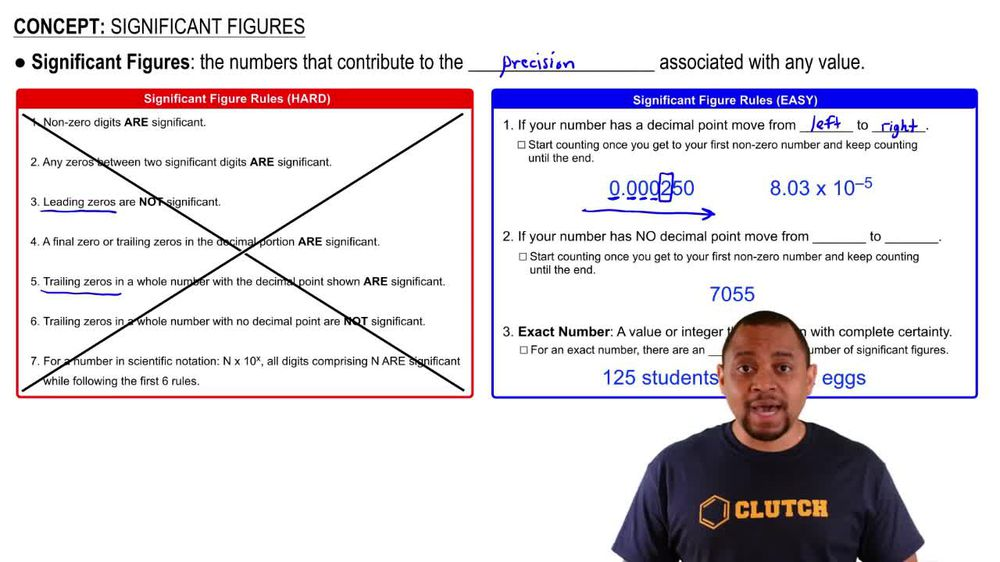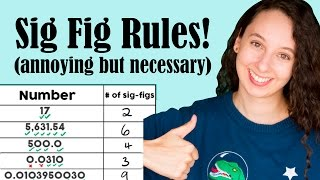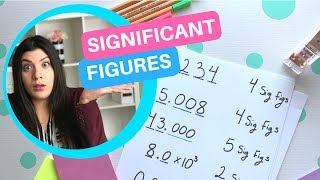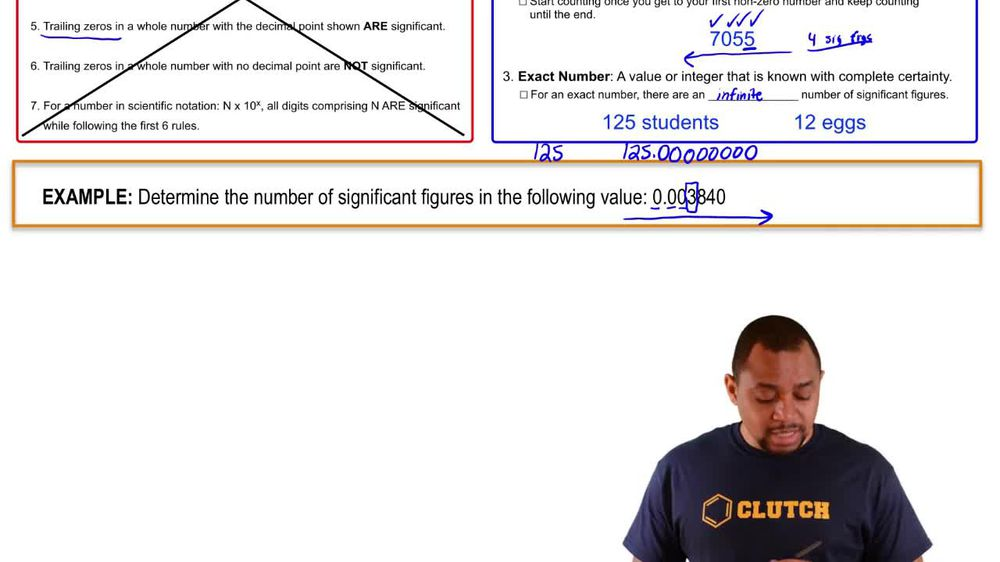Start typing, then use the up and down arrows to select an option from the list.
1. 1. Intro to General Chemistry2. Significant Figures
Problem

# How many significant figures are in each of the following measurements? (e) 5.10 * 102 J

Relevant Solution55s
Play a video:
Hello everyone today. We are being asked to determine how many significant figures there are in 3.46 times 10 to the fourth centimeters squared. An important thing to know about scientific notation in these kinds of problems is that we can simply pretend this 10th of the fourth does not exist and only count the significant figures in the decimal number. In this example we have three non zero integers, 34 and six. Therefore We have a total of three significant figures, and there's our answer. I hope this helped, and until next time.